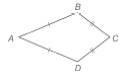Chapter 4.2, Problem 35EElementary Geometry For College St...

7th Edition
Alexander + 2 others
ISBN: 9781337614085

Solutions

Chapter
SectionElementary Geometry For College St...

7th Edition
Alexander + 2 others
ISBN: 9781337614085
Textbook Problem

For Exercises 32 to 35, consider kite A B C D with A B ¯ ≅ A D ¯ and B C ¯ ≅ D C ¯ .For kite A B C D , A B = x 6 + 5 , A D = x 3 + 3 , and B C = x - 2 . Find the perimeter of A B C D .

To determine

To find:

The perimeter of ABCD.

Explanation

Given,

The kite ABCD with

BC¯DC¯,

AB=x6+5,

BC=x-2

In the given kite, we have

Thus,

x6+5=x3+3

Add -x3 on both sides,

x6+5+-x3=x3+3+-x3

x6+-x3+5=x3+-x3+3

x6+-x3+5=3

x6+-x3

Still sussing out bartleby?

Check out a sample textbook solution.

See a sample solution

The Solution to Your Study Problems

Bartleby provides explanations to thousands of textbook problems written by our experts, many with advanced degrees!

Get Started

What is the lowest score in the following distribution?

Essentials of Statistics for The Behavioral Sciences (MindTap Course List)

sinx=ex+ex2

Study Guide for Stewart's Single Variable Calculus: Early Transcendentals, 8th

In Exercises 1-8, determine which of the matrices are regular. 

Finite Mathematics for the Managerial, Life, and Social Sciences